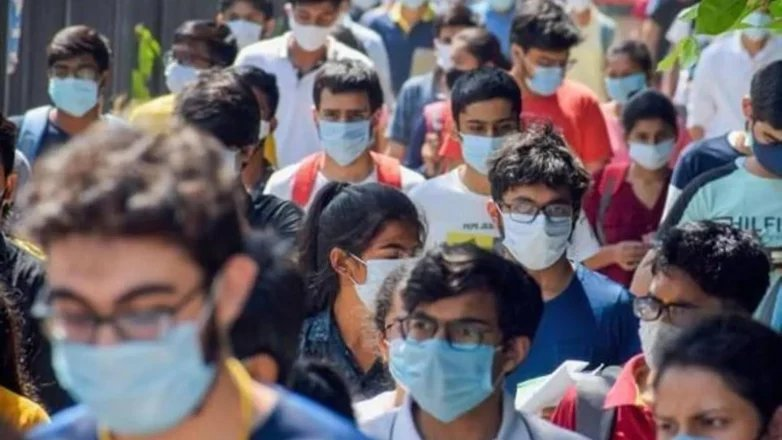By | 28 Aug 2022 at 7:14 PMJEE Advanced 2022 paper analysis: Indian Institute of Technology (IIT) Bombay conducted JEE Advanced 2022 on August 28. Paper 1 was held from 9 am to 12 pm and paper 2 from 2:30 to 5:30 pm. JEE Advanced 2022 live updates.

The paper had 3 parts and 54 questions in total with 18 questions in each part. Maximum marks for each part was 60.

In each part there were three sections-

Sec-I (Max. marks- 24),

Sec-II (Max. Marks- 24)

Sec-III (Max. marks- 12)

Sec-I had Eight questions- with integer type answers, the answer of which was a positive number only.

Marking scheme in this section:

Full marks: +3 for the correct answer

Zero Marks: 0 if unattempted

Negative Marks: -1 in all other cases

Sec-II had Six questions with four options in which ONE OR MORE THAN ONE option(s) were correct.

Marking scheme in this section:

Full marks: +4 If only (all) the correct options are chosen

Partial Marks: +3 If all the four options are correct but ONLY three correct options are chosen

Partial Marks: +2 If three or more options are correct but only two correct options are chosen

Partial Marks: +1 If two or more options are correct but only one correct option is chosen

Zero Marks:: 0 If none of the options are chosen

Negative Marks: -2 In all other cases

Sec-III had Four questions with four options in each in which ONLY ONE of the four options was correct.

Marking scheme in this section:

Full marks: +3 if option chosen is correct

Zero Marks:: 0 if none of the options are chosen

Negative Marks: -1 in all other cases

Immediate reaction of students:

Students found the overall level of paper to be Moderate & Lengthy.

Paper-2 was lengthy but overall easier compared to paper-1 held in the 1st Shift.

Chemistry (Easy). Balanced Section. In Physical Chemistry, there were questions from Electrochemistry, Mole Concept, Surface Chemistry, Thermodynamics & Chemical Kinetics. Organic Chemistry was well distributed with more mixed concept-based questions. No direct questions in Inorganic Chemistry, there were questions from p-block specially reaction based.

Physics (Moderate & Lengthy) There were more concept based and covered all chapters. Students reported this section to be Moderate. Questions were asked from Optics, Kinematics, Work Power & Energy, Rotational Motion, Thermodynamics mixed with Surface Tension, Current Electricity, Electrostatics, Magnetism. Mixed concepts questions took more time, so some students found this section lengthy.

Mathematics (Moderately & Lengthy) There were more questions from Calculus & Coordinate Geometry. Questions were mixed concept questions clubbing two chapters asked from Functions & Progressions, Application of Derivatives clubbed with Differential Equation, Circle clubbed with Trigonometry, Vectors clubbed with Matrices, and some direct questions from Hyperbola, Ellipse, Permutation & Combination, Probability, Definite Integration with Inverse of function. Questions had lengthy calculations.

(Author Ramesh Batlish is Head-FIITJEE Noida. Views expressed here are personal)